# Exploring GAMs with Rosemary Hartman

#### November 9, 2012

Today at Davis R Users’ Group, Rosemary Hartman took us through her work in progress fitting general additive models to organism presence/absence data. Below is her presentation and script. You can get the original script and data here

Also, check the comments below for some discussion of other options for this type of analysis, such as boosted regression trees.

## GAMs using mgcv and amphibian presence/absence dataset
## code and data by Rosemary Hartman (rosehartman at gmail dot com)

## First, load the mgcv package (Simon Wood)
library(mgcv)

## Now, attach your data set (or my data set)

## create a full exploritory model using varibles you think are biologically
## significant (this is pretty subjective, and where you need to use your brain)
## For my data set, I am trying to predict the presence of cascades frogs

fishlakes.logr <- gam(treatment ~ ## "treatment" = breeding cascades frogs in lake
BUBO.breeding + ## breeding toads in lake
count.RACApops.1K +  ##  number of cascades frog populations within 1km
s(silt.total, k=6) + ## amount of silt in lake bed
s(vegetated.area, k=7) + ## total lake area with emergent aquatic vegetation
s(area.10cm, k=6) + ## total lake area less than 10cm deep
s(weighted.dist, k=6) + ## perportion of nearby water bodies with frogs weighted by distance
s(bank.slope, k=6),  ## average slope
family=binomial("logit"), data= fishlakes) ## this is a logistic function since I have binomial data
## Warning: matrix not positive definite
summary(fishlakes.logr) ## Note, this model explains 68.5% of devience
##
## Family: binomial
##
## Formula:
## treatment ~ BUBO.breeding + count.RACApops.1K + s(silt.total,
##     k = 6) + s(vegetated.area, k = 7) + s(area.10cm, k = 6) +
##     s(weighted.dist, k = 6) + s(bank.slope, k = 6)
##
## Parametric coefficients:
##                   Estimate Std. Error z value Pr(>|z|)
## (Intercept)          3.830      3.224    1.19    0.235
## BUBO.breedingy      -3.393      1.646   -2.06    0.039 *
## count.RACApops.1K   -0.192      0.506   -0.38    0.705
## ---
## Signif. codes:  0 '***' 0.001 '**' 0.01 '*' 0.05 '.' 0.1 ' ' 1
##
## Approximate significance of smooth terms:
##                    edf Ref.df Chi.sq p-value
## s(silt.total)     1.00   1.00   0.51    0.47
## s(vegetated.area) 3.48   3.97   3.47    0.48
## s(area.10cm)      2.20   2.66   1.17    0.70
## s(weighted.dist)  2.24   2.73   2.56    0.41
## s(bank.slope)     2.22   2.68   5.02    0.14
##
## R-sq.(adj) =  0.597   Deviance explained = 68.5%
## UBRE score = 0.091416  Scale est. = 1         n = 42
gam.check(fishlakes.logr) ## It seems to think my ks are too low, but raising them
##
## Method: UBRE   Optimizer: outer newton
## full convergence after 14 iterations.
## (score 0.09142 & scale 1).
## Hessian positive definite, eigenvalue range [2.564e-07,0.05689].
##
## Basis dimension (k) checking results. Low p-value (k-index<1) may
## indicate that k is too low, especially if edf is close to k'.
##
##                      k'   edf k-index p-value
## s(silt.total)     5.000 1.000   0.691    0.02
## s(vegetated.area) 6.000 3.485   1.321    0.97
## s(area.10cm)      5.000 2.200   0.742    0.02
## s(weighted.dist)  5.000 2.237   1.064    0.63
## s(bank.slope)     5.000 2.219   0.965    0.42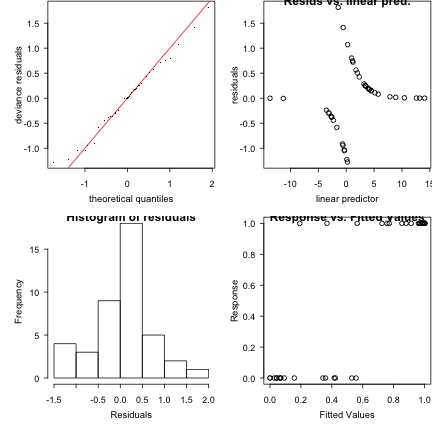## gives huge overspecification. I'm not sure what to do about this.
plot(fishlakes.logr) ## diagnostic plots for the smooths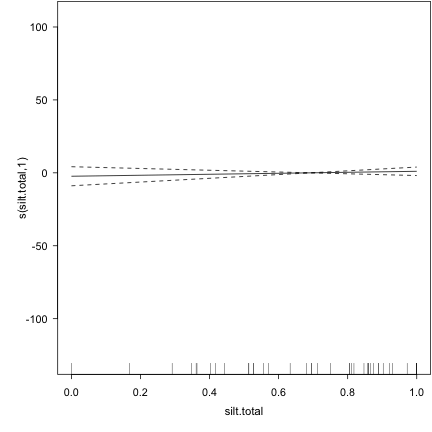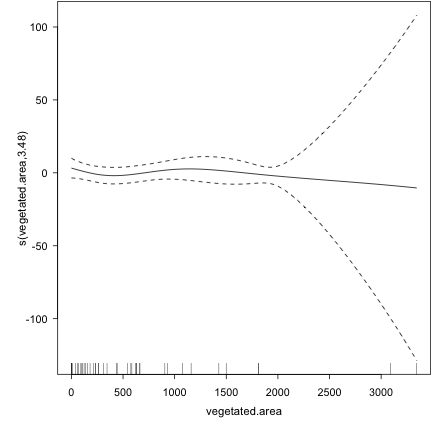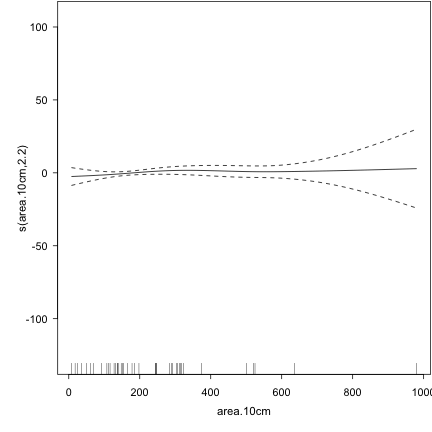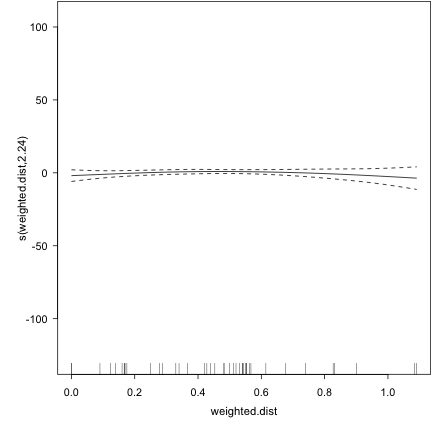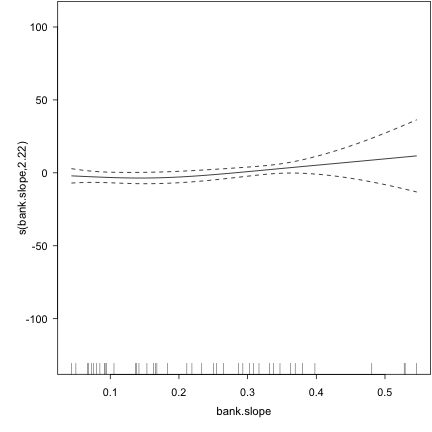## To choose my k's, I looped through a bunch of different values for k, and basically
## just played around until my model stopped overspecifying

for (i in 2:8) { fishlakes.logr <- gam(treatment ~ count.RACApops.1K + BUBO.breeding + s(silt.total, k=i) + s(vegetated.area, k=i) + s(bank.slope, k=i) + s(area.10cm,  k=i) + s(weighted.dist, k=i), family=binomial("logit"), data= fishlakes);
x <- summary(fishlakes.logr);
y <- AIC(fishlakes.logr);
print(i)
print(x)
print(y)
}
## Warning: basis dimension, k, increased to minimum possible

## Warning: basis dimension, k, increased to minimum possible

## Warning: basis dimension, k, increased to minimum possible

## Warning: basis dimension, k, increased to minimum possible

## Warning: basis dimension, k, increased to minimum possible

## Warning: matrix not positive definite

## Warning: matrix not positive definite

## Warning: matrix not positive definite

## Warning: matrix not positive definite

##  2
##
## Family: binomial
##
## Formula:
## treatment ~ count.RACApops.1K + BUBO.breeding + s(silt.total,
##     k = i) + s(vegetated.area, k = i) + s(bank.slope, k = i) +
##     s(area.10cm, k = i) + s(weighted.dist, k = i)
##
## Parametric coefficients:
##                   Estimate Std. Error z value Pr(>|z|)
## (Intercept)          3.011      1.263    2.38    0.017 *
## count.RACApops.1K   -0.247      0.307   -0.80    0.421
## BUBO.breedingy      -2.314      1.010   -2.29    0.022 *
## ---
## Signif. codes:  0 '***' 0.001 '**' 0.01 '*' 0.05 '.' 0.1 ' ' 1
##
## Approximate significance of smooth terms:
##                    edf Ref.df Chi.sq p-value
## s(silt.total)     1.00   1.00   0.05    0.82
## s(vegetated.area) 1.00   1.00   0.27    0.61
## s(bank.slope)     1.63   1.86   1.97    0.34
## s(area.10cm)      1.00   1.00   0.04    0.85
## s(weighted.dist)  1.80   1.96   2.34    0.30
##
## R-sq.(adj) =  0.339   Deviance explained = 42.2%
## UBRE score = 0.21726  Scale est. = 1         n = 42
##  51.12

## Warning: matrix not positive definite

## Warning: matrix not positive definite

## Warning: matrix not positive definite

## Warning: matrix not positive definite

##  3
##
## Family: binomial
##
## Formula:
## treatment ~ count.RACApops.1K + BUBO.breeding + s(silt.total,
##     k = i) + s(vegetated.area, k = i) + s(bank.slope, k = i) +
##     s(area.10cm, k = i) + s(weighted.dist, k = i)
##
## Parametric coefficients:
##                   Estimate Std. Error z value Pr(>|z|)
## (Intercept)          3.011      1.263    2.38    0.017 *
## count.RACApops.1K   -0.247      0.307   -0.80    0.421
## BUBO.breedingy      -2.314      1.010   -2.29    0.022 *
## ---
## Signif. codes:  0 '***' 0.001 '**' 0.01 '*' 0.05 '.' 0.1 ' ' 1
##
## Approximate significance of smooth terms:
##                    edf Ref.df Chi.sq p-value
## s(silt.total)     1.00   1.00   0.05    0.82
## s(vegetated.area) 1.00   1.00   0.27    0.61
## s(bank.slope)     1.63   1.86   1.97    0.34
## s(area.10cm)      1.00   1.00   0.04    0.85
## s(weighted.dist)  1.80   1.96   2.34    0.30
##
## R-sq.(adj) =  0.339   Deviance explained = 42.2%
## UBRE score = 0.21726  Scale est. = 1         n = 42
##  51.12

## Warning: matrix not positive definite

## Warning: matrix not positive definite

## Warning: matrix not positive definite

## Warning: matrix not positive definite

##  4
##
## Family: binomial
##
## Formula:
## treatment ~ count.RACApops.1K + BUBO.breeding + s(silt.total,
##     k = i) + s(vegetated.area, k = i) + s(bank.slope, k = i) +
##     s(area.10cm, k = i) + s(weighted.dist, k = i)
##
## Parametric coefficients:
##                   Estimate Std. Error z value Pr(>|z|)
## (Intercept)          2.745      1.147    2.39    0.017 *
## count.RACApops.1K   -0.217      0.299   -0.73    0.468
## BUBO.breedingy      -2.308      0.996   -2.32    0.020 *
## ---
## Signif. codes:  0 '***' 0.001 '**' 0.01 '*' 0.05 '.' 0.1 ' ' 1
##
## Approximate significance of smooth terms:
##                    edf Ref.df Chi.sq p-value
## s(silt.total)     1.00   1.00   0.06    0.81
## s(vegetated.area) 1.00   1.00   0.31    0.58
## s(bank.slope)     1.77   2.14   2.38    0.33
## s(area.10cm)      1.00   1.00   0.08    0.78
## s(weighted.dist)  1.86   2.25   2.53    0.32
##
## R-sq.(adj) =  0.332   Deviance explained =   42%
## UBRE score = 0.22873  Scale est. = 1         n = 42
##  51.61

## Warning: matrix not positive definite

## Warning: matrix not positive definite

## Warning: matrix not positive definite

##  5
##
## Family: binomial
##
## Formula:
## treatment ~ count.RACApops.1K + BUBO.breeding + s(silt.total,
##     k = i) + s(vegetated.area, k = i) + s(bank.slope, k = i) +
##     s(area.10cm, k = i) + s(weighted.dist, k = i)
##
## Parametric coefficients:
##                   Estimate Std. Error z value Pr(>|z|)
## (Intercept)          -16.5     4857.1    0.00     1.00
## count.RACApops.1K     42.4      397.8    0.11     0.92
## BUBO.breedingy      -254.6     9152.1   -0.03     0.98
##
## Approximate significance of smooth terms:
##                    edf Ref.df Chi.sq p-value
## s(silt.total)     1.00   1.00   0.00    0.95
## s(vegetated.area) 2.32   2.36   0.00    1.00
## s(bank.slope)     1.52   1.57   0.01    0.99
## s(area.10cm)      3.06   3.07   0.02    1.00
## s(weighted.dist)  4.00   4.00   0.02    1.00
##
## R-sq.(adj) =      1   Deviance explained =  100%
## UBRE score = -0.29037  Scale est. = 1         n = 42
##  29.8

## Warning: matrix not positive definite

##  6
##
## Family: binomial
##
## Formula:
## treatment ~ count.RACApops.1K + BUBO.breeding + s(silt.total,
##     k = i) + s(vegetated.area, k = i) + s(bank.slope, k = i) +
##     s(area.10cm, k = i) + s(weighted.dist, k = i)
##
## Parametric coefficients:
##                   Estimate Std. Error z value Pr(>|z|)
## (Intercept)         5.4778     2.5608    2.14    0.032 *
## count.RACApops.1K  -0.0673     0.4763   -0.14    0.888
## BUBO.breedingy     -4.9379     2.1801   -2.26    0.024 *
## ---
## Signif. codes:  0 '***' 0.001 '**' 0.01 '*' 0.05 '.' 0.1 ' ' 1
##
## Approximate significance of smooth terms:
##                    edf Ref.df Chi.sq p-value
## s(silt.total)     1.00   1.00   0.14    0.71
## s(vegetated.area) 1.00   1.00   0.38    0.54
## s(bank.slope)     2.14   2.60   3.51    0.25
## s(area.10cm)      5.00   5.00   5.46    0.36
## s(weighted.dist)  2.13   2.64   2.50    0.40
##
## R-sq.(adj) =  0.523   Deviance explained = 65.4%
## UBRE score = 0.13954  Scale est. = 1         n = 42
##  47.86
##  7
##
## Family: binomial
##
## Formula:
## treatment ~ count.RACApops.1K + BUBO.breeding + s(silt.total,
##     k = i) + s(vegetated.area, k = i) + s(bank.slope, k = i) +
##     s(area.10cm, k = i) + s(weighted.dist, k = i)
##
## Parametric coefficients:
##                   Estimate Std. Error z value Pr(>|z|)
## (Intercept)           6.56     366.37    0.02     0.99
## count.RACApops.1K     4.30      13.89    0.31     0.76
## BUBO.breedingy      -22.94      36.65   -0.63     0.53
##
## Approximate significance of smooth terms:
##                    edf Ref.df Chi.sq p-value
## s(silt.total)     1.00   1.00   0.02    0.88
## s(vegetated.area) 3.50   3.82   0.97    0.90
## s(bank.slope)     1.00   1.00   0.13    0.72
## s(area.10cm)      1.00   1.00   0.61    0.44
## s(weighted.dist)  4.02   4.21   1.32    0.88
##
## R-sq.(adj) =      1   Deviance explained = 99.7%
## UBRE score = -0.35283  Scale est. = 1         n = 42
##  27.18

## Warning: matrix not positive definite

## Warning: matrix not positive definite

## Warning: matrix not positive definite

## Warning: matrix not positive definite

##  8
##
## Family: binomial
##
## Formula:
## treatment ~ count.RACApops.1K + BUBO.breeding + s(silt.total,
##     k = i) + s(vegetated.area, k = i) + s(bank.slope, k = i) +
##     s(area.10cm, k = i) + s(weighted.dist, k = i)
##
## Parametric coefficients:
##                   Estimate Std. Error z value Pr(>|z|)
## (Intercept)         22.752    135.686    0.17     0.87
## count.RACApops.1K   -0.247     23.133   -0.01     0.99
## BUBO.breedingy     -29.877     67.934   -0.44     0.66
##
## Approximate significance of smooth terms:
##                    edf Ref.df Chi.sq p-value
## s(silt.total)     4.58   4.71   0.09    1.00
## s(vegetated.area) 3.19   3.31   0.01    1.00
## s(bank.slope)     1.00   1.00   0.06    0.80
## s(area.10cm)      2.14   2.23   0.02    0.99
## s(weighted.dist)  3.68   3.87   0.04    1.00
##
## R-sq.(adj) =      1   Deviance explained = 99.9%
## UBRE score = -0.16157  Scale est. = 1         n = 42
##  35.21

for (i in 2:10) { fishlakes.logr <- gam(treatment ~ count.RACApops.1K + BUBO.breeding + s(silt.total, k=i) + s(vegetated.area, k=7) + s(bank.slope, k=6) + s(area.10cm,  k=6) + s(weighted.dist, k=6), family=binomial("logit"), data= fishlakes);
x <- summary(fishlakes.logr);
y <- AIC(fishlakes.logr);
print(i)
print(x)
print(y)
}
## Warning: basis dimension, k, increased to minimum possible

## Warning: matrix not positive definite

##  2
##
## Family: binomial
##
## Formula:
## treatment ~ count.RACApops.1K + BUBO.breeding + s(silt.total,
##     k = i) + s(vegetated.area, k = 7) + s(bank.slope, k = 6) +
##     s(area.10cm, k = 6) + s(weighted.dist, k = 6)
##
## Parametric coefficients:
##                   Estimate Std. Error z value Pr(>|z|)
## (Intercept)         5.4778     2.5608    2.14    0.032 *
## count.RACApops.1K  -0.0673     0.4763   -0.14    0.888
## BUBO.breedingy     -4.9379     2.1801   -2.26    0.024 *
## ---
## Signif. codes:  0 '***' 0.001 '**' 0.01 '*' 0.05 '.' 0.1 ' ' 1
##
## Approximate significance of smooth terms:
##                    edf Ref.df Chi.sq p-value
## s(silt.total)     1.00   1.00   0.14    0.71
## s(vegetated.area) 1.00   1.00   0.38    0.54
## s(bank.slope)     2.14   2.60   3.51    0.25
## s(area.10cm)      5.00   5.00   5.46    0.36
## s(weighted.dist)  2.13   2.64   2.50    0.40
##
## R-sq.(adj) =  0.523   Deviance explained = 65.4%
## UBRE score = 0.13954  Scale est. = 1         n = 42
##  47.86

## Warning: matrix not positive definite

##  3
##
## Family: binomial
##
## Formula:
## treatment ~ count.RACApops.1K + BUBO.breeding + s(silt.total,
##     k = i) + s(vegetated.area, k = 7) + s(bank.slope, k = 6) +
##     s(area.10cm, k = 6) + s(weighted.dist, k = 6)
##
## Parametric coefficients:
##                   Estimate Std. Error z value Pr(>|z|)
## (Intercept)         5.4778     2.5608    2.14    0.032 *
## count.RACApops.1K  -0.0673     0.4763   -0.14    0.888
## BUBO.breedingy     -4.9379     2.1801   -2.26    0.024 *
## ---
## Signif. codes:  0 '***' 0.001 '**' 0.01 '*' 0.05 '.' 0.1 ' ' 1
##
## Approximate significance of smooth terms:
##                    edf Ref.df Chi.sq p-value
## s(silt.total)     1.00   1.00   0.14    0.71
## s(vegetated.area) 1.00   1.00   0.38    0.54
## s(bank.slope)     2.14   2.60   3.51    0.25
## s(area.10cm)      5.00   5.00   5.46    0.36
## s(weighted.dist)  2.13   2.64   2.50    0.40
##
## R-sq.(adj) =  0.523   Deviance explained = 65.4%
## UBRE score = 0.13954  Scale est. = 1         n = 42
##  47.86
##  4
##
## Family: binomial
##
## Formula:
## treatment ~ count.RACApops.1K + BUBO.breeding + s(silt.total,
##     k = i) + s(vegetated.area, k = 7) + s(bank.slope, k = 6) +
##     s(area.10cm, k = 6) + s(weighted.dist, k = 6)
##
## Parametric coefficients:
##                   Estimate Std. Error z value Pr(>|z|)
## (Intercept)           76.6      427.3    0.18     0.86
## count.RACApops.1K     10.1       51.7    0.20     0.84
## BUBO.breedingy       -89.3      536.3   -0.17     0.87
##
## Approximate significance of smooth terms:
##                    edf Ref.df Chi.sq p-value
## s(silt.total)     1.00   1.00   0.00    0.97
## s(vegetated.area) 2.65   2.70   0.03    1.00
## s(bank.slope)     3.77   3.87   0.12    1.00
## s(area.10cm)      4.51   4.54   0.19    1.00
## s(weighted.dist)  1.87   1.90   0.03    0.98
##
## R-sq.(adj) =      1   Deviance explained =  100%
## UBRE score = -0.19934  Scale est. = 1         n = 42
##  33.63

## Warning: matrix not positive definite

##  5
##
## Family: binomial
##
## Formula:
## treatment ~ count.RACApops.1K + BUBO.breeding + s(silt.total,
##     k = i) + s(vegetated.area, k = 7) + s(bank.slope, k = 6) +
##     s(area.10cm, k = 6) + s(weighted.dist, k = 6)
##
## Parametric coefficients:
##                   Estimate Std. Error z value Pr(>|z|)
## (Intercept)         5.4778     2.5609    2.14    0.032 *
## count.RACApops.1K  -0.0673     0.4763   -0.14    0.888
## BUBO.breedingy     -4.9379     2.1801   -2.26    0.024 *
## ---
## Signif. codes:  0 '***' 0.001 '**' 0.01 '*' 0.05 '.' 0.1 ' ' 1
##
## Approximate significance of smooth terms:
##                    edf Ref.df Chi.sq p-value
## s(silt.total)     1.00   1.00   0.14    0.71
## s(vegetated.area) 1.00   1.00   0.38    0.54
## s(bank.slope)     2.14   2.60   3.51    0.25
## s(area.10cm)      5.00   5.00   5.46    0.36
## s(weighted.dist)  2.13   2.64   2.50    0.40
##
## R-sq.(adj) =  0.523   Deviance explained = 65.4%
## UBRE score = 0.13954  Scale est. = 1         n = 42
##  47.86

## Warning: matrix not positive definite

##  6
##
## Family: binomial
##
## Formula:
## treatment ~ count.RACApops.1K + BUBO.breeding + s(silt.total,
##     k = i) + s(vegetated.area, k = 7) + s(bank.slope, k = 6) +
##     s(area.10cm, k = 6) + s(weighted.dist, k = 6)
##
## Parametric coefficients:
##                   Estimate Std. Error z value Pr(>|z|)
## (Intercept)          3.830      3.224    1.19    0.235
## count.RACApops.1K   -0.192      0.506   -0.38    0.705
## BUBO.breedingy      -3.393      1.646   -2.06    0.039 *
## ---
## Signif. codes:  0 '***' 0.001 '**' 0.01 '*' 0.05 '.' 0.1 ' ' 1
##
## Approximate significance of smooth terms:
##                    edf Ref.df Chi.sq p-value
## s(silt.total)     1.00   1.00   0.51    0.47
## s(vegetated.area) 3.48   3.97   3.47    0.48
## s(bank.slope)     2.22   2.68   4.07    0.21
## s(area.10cm)      2.20   2.66   1.09    0.72
## s(weighted.dist)  2.24   2.73   1.90    0.54
##
## R-sq.(adj) =  0.597   Deviance explained = 68.5%
## UBRE score = 0.091416  Scale est. = 1         n = 42
##  45.84
##  7
##
## Family: binomial
##
## Formula:
## treatment ~ count.RACApops.1K + BUBO.breeding + s(silt.total,
##     k = i) + s(vegetated.area, k = 7) + s(bank.slope, k = 6) +
##     s(area.10cm, k = 6) + s(weighted.dist, k = 6)
##
## Parametric coefficients:
##                   Estimate Std. Error z value Pr(>|z|)
## (Intercept)          55.26     358.87    0.15     0.88
## count.RACApops.1K     5.42      99.67    0.05     0.96
## BUBO.breedingy      -87.21     624.65   -0.14     0.89
##
## Approximate significance of smooth terms:
##                    edf Ref.df Chi.sq p-value
## s(silt.total)     4.96   4.99   0.09    1.00
## s(vegetated.area) 3.99   4.02   0.02    1.00
## s(bank.slope)     1.00   1.00   0.01    0.93
## s(area.10cm)      1.86   1.96   0.02    0.99
## s(weighted.dist)  1.03   1.03   0.01    0.92
##
## R-sq.(adj) =      1   Deviance explained =  100%
## UBRE score = -0.24525  Scale est. = 1         n = 42
##  31.7

## Warning: matrix not positive definite

##  8
##
## Family: binomial
##
## Formula:
## treatment ~ count.RACApops.1K + BUBO.breeding + s(silt.total,
##     k = i) + s(vegetated.area, k = 7) + s(bank.slope, k = 6) +
##     s(area.10cm, k = 6) + s(weighted.dist, k = 6)
##
## Parametric coefficients:
##                   Estimate Std. Error z value Pr(>|z|)
## (Intercept)          89.75   12140.84    0.01     0.99
## count.RACApops.1K    -3.53    1710.88    0.00     1.00
## BUBO.breedingy     -119.62    9443.72   -0.01     0.99
##
## Approximate significance of smooth terms:
##                    edf Ref.df Chi.sq p-value
## s(silt.total)     5.76   5.80      0       1
## s(vegetated.area) 2.03   2.04      0       1
## s(bank.slope)     1.18   1.19      0       1
## s(area.10cm)      1.84   1.90      0       1
## s(weighted.dist)  1.08   1.08      0       1
##
## R-sq.(adj) =      1   Deviance explained =  100%
## UBRE score = -0.29122  Scale est. = 1         n = 42
##  29.77
##  9
##
## Family: binomial
##
## Formula:
## treatment ~ count.RACApops.1K + BUBO.breeding + s(silt.total,
##     k = i) + s(vegetated.area, k = 7) + s(bank.slope, k = 6) +
##     s(area.10cm, k = 6) + s(weighted.dist, k = 6)
##
## Parametric coefficients:
##                   Estimate Std. Error z value Pr(>|z|)
## (Intercept)         48.947    655.719    0.07     0.94
## count.RACApops.1K   -0.137     82.969    0.00     1.00
## BUBO.breedingy     -73.934    546.328   -0.14     0.89
##
## Approximate significance of smooth terms:
##                    edf Ref.df Chi.sq p-value
## s(silt.total)     5.75   5.84   0.02    1.00
## s(vegetated.area) 2.00   2.01   0.01    1.00
## s(bank.slope)     1.00   1.00   0.01    0.91
## s(area.10cm)      1.86   1.95   0.01    0.99
## s(weighted.dist)  1.19   1.21   0.00    0.99
##
## R-sq.(adj) =      1   Deviance explained =  100%
## UBRE score = -0.29492  Scale est. = 1         n = 42
##  29.61
##  10
##
## Family: binomial
##
## Formula:
## treatment ~ count.RACApops.1K + BUBO.breeding + s(silt.total,
##     k = i) + s(vegetated.area, k = 7) + s(bank.slope, k = 6) +
##     s(area.10cm, k = 6) + s(weighted.dist, k = 6)
##
## Parametric coefficients:
##                   Estimate Std. Error z value Pr(>|z|)
## (Intercept)        54.2952   102.0865    0.53     0.59
## count.RACApops.1K  -0.0411    16.5558    0.00     1.00
## BUBO.breedingy    -75.4705   126.5539   -0.60     0.55
##
## Approximate significance of smooth terms:
##                    edf Ref.df Chi.sq p-value
## s(silt.total)     6.88   7.06   0.74    1.00
## s(vegetated.area) 3.09   3.16   0.22    0.98
## s(bank.slope)     1.04   1.05   0.22    0.66
## s(area.10cm)      2.42   2.67   0.38    0.92
## s(weighted.dist)  1.00   1.00   0.30    0.58
##
## R-sq.(adj) =      1   Deviance explained = 99.8%
## UBRE score = -0.16704  Scale est. = 1         n = 42
##  34.98

## In order to see which of the candidate terms are significant, I will run the model
## several times, dropping one term out each time and then do a likelyhood ratio test
## to see if adding the term leads to a significant improvement in residual deviance.

## fishlakes2.logr is the same model as fishlakes.logr without the "bank slope" term
fishlakes2.logr <- gam(treatment ~ BUBO.breeding + count.RACApops.1K +
s(silt.total, k=6) + s(vegetated.area, k=7) + s(area.10cm, k=6) + s(weighted.dist, k=6),
family=binomial("logit"), data= fishlakes)
## Warning: matrix not positive definite
summary(fishlakes2.logr) ## This model only explains 34.1% of deviance
##
## Family: binomial
##
## Formula:
## treatment ~ BUBO.breeding + count.RACApops.1K + s(silt.total,
##     k = 6) + s(vegetated.area, k = 7) + s(area.10cm, k = 6) +
##     s(weighted.dist, k = 6)
##
## Parametric coefficients:
##                   Estimate Std. Error z value Pr(>|z|)
## (Intercept)          2.175      0.974    2.23    0.026 *
## BUBO.breedingy      -1.840      0.891   -2.07    0.039 *
## count.RACApops.1K   -0.294      0.290   -1.01    0.311
## ---
## Signif. codes:  0 '***' 0.001 '**' 0.01 '*' 0.05 '.' 0.1 ' ' 1
##
## Approximate significance of smooth terms:
##                    edf Ref.df Chi.sq p-value
## s(silt.total)     1.00   1.00   3.19   0.074 .
## s(vegetated.area) 1.00   1.00   0.46   0.499
## s(area.10cm)      1.00   1.00   1.82   0.177
## s(weighted.dist)  2.02   2.54   2.53   0.375
## ---
## Signif. codes:  0 '***' 0.001 '**' 0.01 '*' 0.05 '.' 0.1 ' ' 1
##
## R-sq.(adj) =  0.279   Deviance explained = 34.1%
## UBRE score = 0.25725  Scale est. = 1         n = 42
anova(fishlakes2.logr,  fishlakes.logr,  test = "LRT") ## There is a significant difference
## Analysis of Deviance Table
##
## Model 1: treatment ~ BUBO.breeding + count.RACApops.1K + s(silt.total,
##     k = 6) + s(vegetated.area, k = 7) + s(area.10cm, k = 6) +
##     s(weighted.dist, k = 6)
## Model 2: treatment ~ count.RACApops.1K + BUBO.breeding + s(silt.total,
##     k = i) + s(vegetated.area, k = 7) + s(bank.slope, k = 6) +
##     s(area.10cm, k = 6) + s(weighted.dist, k = 6)
##   Resid. Df Resid. Dev   Df Deviance Pr(>Chi)
## 1      34.0       36.8
## 2      24.6        0.1 9.41     36.6  4.2e-05 ***
## ---
## Signif. codes:  0 '***' 0.001 '**' 0.01 '*' 0.05 '.' 0.1 ' ' 1 
## in explanitory power between the two models, that means the "bank slope" term
## should be included in the final model

## Now do the same with the rest of the varibles
fishlakes3.logr <- gam(treatment ~ BUBO.breeding + count.RACApops.1K +s(silt.total, k=6)
+ s(vegetated.area, k=7) + s(bank.slope, k=6) + s(area.10cm, k=6),
family=binomial("logit"), data= fishlakes) ## all variables except "weighted distance"
## Warning: matrix not positive definite
summary(fishlakes3.logr) ## This model is way overspecified (100% of devience explained)
##
## Family: binomial
##
## Formula:
## treatment ~ BUBO.breeding + count.RACApops.1K + s(silt.total,
##     k = 6) + s(vegetated.area, k = 7) + s(bank.slope, k = 6) +
##     s(area.10cm, k = 6)
##
## Parametric coefficients:
##                   Estimate Std. Error z value Pr(>|z|)
## (Intercept)          77.43     282.28    0.27     0.78
## BUBO.breedingy     -120.75     510.54   -0.24     0.81
## count.RACApops.1K     2.83      64.25    0.04     0.96
##
## Approximate significance of smooth terms:
##                    edf Ref.df Chi.sq p-value
## s(silt.total)     4.77   4.80   0.08    1.00
## s(vegetated.area) 2.15   2.19   0.02    0.99
## s(bank.slope)     1.22   1.25   0.06    0.88
## s(area.10cm)      3.71   3.77   0.06    1.00
##
## R-sq.(adj) =      1   Deviance explained =  100%
## UBRE score = -0.29299  Scale est. = 1         n = 42
anova(fishlakes3.logr,  fishlakes.logr,  test = "LRT") ## significant differnce!
## Analysis of Deviance Table
##
## Model 1: treatment ~ BUBO.breeding + count.RACApops.1K + s(silt.total,
##     k = 6) + s(vegetated.area, k = 7) + s(bank.slope, k = 6) +
##     s(area.10cm, k = 6)
## Model 2: treatment ~ count.RACApops.1K + BUBO.breeding + s(silt.total,
##     k = i) + s(vegetated.area, k = 7) + s(bank.slope, k = 6) +
##     s(area.10cm, k = 6) + s(weighted.dist, k = 6)
##   Resid. Df Resid. Dev   Df Deviance Pr(>Chi)
## 1      27.2      0.008
## 2      24.6      0.127 2.59   -0.119         
## Include "weighted distance" in final model

fishlakes4.logr <- gam(treatment ~ BUBO.breeding +count.RACApops.1K+
s(silt.total, k=6) + s(vegetated.area, k=7) +
s(bank.slope, k=6) + s(weighted.dist, k=6),
family=binomial("logit"), data= fishlakes) ## all variables except area under 10cm
## Warning: matrix not positive definite
summary(fishlakes4.logr) ## This model only explains 54.5% of deviance
##
## Family: binomial
##
## Formula:
## treatment ~ BUBO.breeding + count.RACApops.1K + s(silt.total,
##     k = 6) + s(vegetated.area, k = 7) + s(bank.slope, k = 6) +
##     s(weighted.dist, k = 6)
##
## Parametric coefficients:
##                   Estimate Std. Error z value Pr(>|z|)
## (Intercept)          2.490      1.851    1.35    0.179
## BUBO.breedingy      -1.997      1.100   -1.82    0.069 .
## count.RACApops.1K   -0.465      0.373   -1.25    0.213
## ---
## Signif. codes:  0 '***' 0.001 '**' 0.01 '*' 0.05 '.' 0.1 ' ' 1
##
## Approximate significance of smooth terms:
##                    edf Ref.df Chi.sq p-value
## s(silt.total)     1.00   1.00   0.03   0.852
## s(vegetated.area) 3.29   3.81   4.86   0.276
## s(bank.slope)     1.00   1.00   4.12   0.042 *
## s(weighted.dist)  1.40   1.70   0.81   0.591
## ---
## Signif. codes:  0 '***' 0.001 '**' 0.01 '*' 0.05 '.' 0.1 ' ' 1
##
## R-sq.(adj) =  0.513   Deviance explained = 54.5%
## UBRE score = 0.066475  Scale est. = 1         n = 42
anova(fishlakes4.logr,  fishlakes.logr,  test = "LRT") ## the difference is not
## Analysis of Deviance Table
##
## Model 1: treatment ~ BUBO.breeding + count.RACApops.1K + s(silt.total,
##     k = 6) + s(vegetated.area, k = 7) + s(bank.slope, k = 6) +
##     s(weighted.dist, k = 6)
## Model 2: treatment ~ count.RACApops.1K + BUBO.breeding + s(silt.total,
##     k = i) + s(vegetated.area, k = 7) + s(bank.slope, k = 6) +
##     s(area.10cm, k = 6) + s(weighted.dist, k = 6)
##   Resid. Df Resid. Dev   Df Deviance Pr(>Chi)
## 1      32.3      25.40
## 2      24.6       0.13 7.73     25.3   0.0012 **
## ---
## Signif. codes:  0 '***' 0.001 '**' 0.01 '*' 0.05 '.' 0.1 ' ' 1 
## significant, leave "area under 10cm" out of final model

fishlakes5.logr <- gam(treatment ~ BUBO.breeding +
s(silt.total, k=6) + s(vegetated.area, k=7)+ s(area.10cm, k=6) +
s(weighted.dist, k=6), family=binomial("logit"), data= fishlakes) ## all variables except number
## Warning: matrix not positive definite
## of frog populations within 1km
summary(fishlakes5.logr) ## This model only explains 31.8% of deviance
##
## Family: binomial
##
## Formula:
## treatment ~ BUBO.breeding + s(silt.total, k = 6) + s(vegetated.area,
##     k = 7) + s(area.10cm, k = 6) + s(weighted.dist, k = 6)
##
## Parametric coefficients:
##                Estimate Std. Error z value Pr(>|z|)
## (Intercept)       1.529      0.677    2.26    0.024 *
## BUBO.breedingy   -1.608      0.836   -1.92    0.054 .
## ---
## Signif. codes:  0 '***' 0.001 '**' 0.01 '*' 0.05 '.' 0.1 ' ' 1
##
## Approximate significance of smooth terms:
##                    edf Ref.df Chi.sq p-value
## s(silt.total)     1.00   1.00   2.76   0.097 .
## s(vegetated.area) 1.00   1.00   0.30   0.583
## s(area.10cm)      1.00   1.00   1.56   0.212
## s(weighted.dist)  1.94   2.45   2.26   0.403
## ---
## Signif. codes:  0 '***' 0.001 '**' 0.01 '*' 0.05 '.' 0.1 ' ' 1
##
## R-sq.(adj) =   0.26   Deviance explained = 31.8%
## UBRE score = 0.23669  Scale est. = 1         n = 42
anova(fishlakes5.logr,  fishlakes.logr,  test = "LRT") ## significant differnce!
## Analysis of Deviance Table
##
## Model 1: treatment ~ BUBO.breeding + s(silt.total, k = 6) + s(vegetated.area,
##     k = 7) + s(area.10cm, k = 6) + s(weighted.dist, k = 6)
## Model 2: treatment ~ count.RACApops.1K + BUBO.breeding + s(silt.total,
##     k = i) + s(vegetated.area, k = 7) + s(bank.slope, k = 6) +
##     s(area.10cm, k = 6) + s(weighted.dist, k = 6)
##   Resid. Df Resid. Dev   Df Deviance Pr(>Chi)
## 1      35.1       38.1
## 2      24.6        0.1 10.5     37.9  5.6e-05 ***
## ---
## Signif. codes:  0 '***' 0.001 '**' 0.01 '*' 0.05 '.' 0.1 ' ' 1 
## Include "count RACA pops" in final model

fishlakes6.logr <-gam(treatment ~ BUBO.breeding + count.RACApops.1K+
s(silt.total, k=6) + s(bank.slope, k=6) + s(area.10cm, k=6) + s(weighted.dist, k=6),
family=binomial("logit"), data= fishlakes) ## all varibles except vegetated area
## Warning: matrix not positive definite
summary(fishlakes6.logr) ## This model explains 61.4% of deviance 
##
## Family: binomial
##
## Formula:
## treatment ~ BUBO.breeding + count.RACApops.1K + s(silt.total,
##     k = 6) + s(bank.slope, k = 6) + s(area.10cm, k = 6) + s(weighted.dist,
##     k = 6)
##
## Parametric coefficients:
##                   Estimate Std. Error z value Pr(>|z|)
## (Intercept)          3.967      1.717    2.31    0.021 *
## BUBO.breedingy      -3.711      1.561   -2.38    0.017 *
## count.RACApops.1K   -0.085      0.412   -0.21    0.836
## ---
## Signif. codes:  0 '***' 0.001 '**' 0.01 '*' 0.05 '.' 0.1 ' ' 1
##
## Approximate significance of smooth terms:
##                   edf Ref.df Chi.sq p-value
## s(silt.total)    1.00   1.00   0.01    0.91
## s(bank.slope)    1.90   2.32   3.93    0.18
## s(area.10cm)     4.67   4.94   6.03    0.30
## s(weighted.dist) 1.93   2.38   2.05    0.43
##
## R-sq.(adj) =  0.512   Deviance explained = 61.4%
## UBRE score = 0.10827  Scale est. = 1         n = 42
anova(fishlakes6.logr,  fishlakes.logr,  test = "LRT") ## Only marginally significant
## Analysis of Deviance Table
##
## Model 1: treatment ~ BUBO.breeding + count.RACApops.1K + s(silt.total,
##     k = 6) + s(bank.slope, k = 6) + s(area.10cm, k = 6) + s(weighted.dist,
##     k = 6)
## Model 2: treatment ~ count.RACApops.1K + BUBO.breeding + s(silt.total,
##     k = i) + s(vegetated.area, k = 7) + s(bank.slope, k = 6) +
##     s(area.10cm, k = 6) + s(weighted.dist, k = 6)
##   Resid. Df Resid. Dev   Df Deviance Pr(>Chi)
## 1      29.5      21.56
## 2      24.6       0.13 4.94     21.4  0.00063 ***
## ---
## Signif. codes:  0 '***' 0.001 '**' 0.01 '*' 0.05 '.' 0.1 ' ' 1 
## maybe drop vegetated area out of final model

fishlakes7.logr <- gam(treatment ~ BUBO.breeding + count.RACApops.1K+  s(vegetated.area, k=7) + s(bank.slope, k=6) + s(area.10cm, k=6) +
s(weighted.dist, k=6), family=binomial("logit"), data= fishlakes) ## all variables except silt
summary(fishlakes7.logr) ## This model explains 63.3% of deviance 
##
## Family: binomial
##
## Formula:
## treatment ~ BUBO.breeding + count.RACApops.1K + s(vegetated.area,
##     k = 7) + s(bank.slope, k = 6) + s(area.10cm, k = 6) + s(weighted.dist,
##     k = 6)
##
## Parametric coefficients:
##                   Estimate Std. Error z value Pr(>|z|)
## (Intercept)         4.6494     2.0998    2.21    0.027 *
## BUBO.breedingy     -4.2713     1.8364   -2.33    0.020 *
## count.RACApops.1K  -0.0772     0.4423   -0.17    0.862
## ---
## Signif. codes:  0 '***' 0.001 '**' 0.01 '*' 0.05 '.' 0.1 ' ' 1
##
## Approximate significance of smooth terms:
##                    edf Ref.df Chi.sq p-value
## s(vegetated.area) 1.00   1.00   0.14   0.707
## s(bank.slope)     2.01   2.46   6.56   0.058 .
## s(area.10cm)      4.87   4.98   5.87   0.317
## s(weighted.dist)  1.99   2.44   2.23   0.410
## ---
## Signif. codes:  0 '***' 0.001 '**' 0.01 '*' 0.05 '.' 0.1 ' ' 1
##
## R-sq.(adj) =  0.524   Deviance explained = 63.3%
## UBRE score = 0.10056  Scale est. = 1         n = 42
anova(fishlakes7.logr,  fishlakes.logr,  test = "LRT") ## No difference. Definitely
## Analysis of Deviance Table
##
## Model 1: treatment ~ BUBO.breeding + count.RACApops.1K + s(vegetated.area,
##     k = 7) + s(bank.slope, k = 6) + s(area.10cm, k = 6) + s(weighted.dist,
##     k = 6)
## Model 2: treatment ~ count.RACApops.1K + BUBO.breeding + s(silt.total,
##     k = i) + s(vegetated.area, k = 7) + s(bank.slope, k = 6) +
##     s(area.10cm, k = 6) + s(weighted.dist, k = 6)
##   Resid. Df Resid. Dev   Df Deviance Pr(>Chi)
## 1      29.1      20.50
## 2      24.6       0.13 4.56     20.4  0.00072 ***
## ---
## Signif. codes:  0 '***' 0.001 '**' 0.01 '*' 0.05 '.' 0.1 ' ' 1 
## drop silt out of final model

fishlakes8.logr <- gam(treatment ~ count.RACApops.1K+ s(vegetated.area, k=7) + s(silt.total, k=6) +
s(bank.slope, k=5) + s(area.10cm, k=5) + s(weighted.dist, k=5),
family=binomial("logit"), data= fishlakes) ## all variables except toads
## Warning: matrix not positive definite

## Warning: matrix not positive definite

## Warning: matrix not positive definite
summary(fishlakes8.logr) ## This model only explains 49.7% of deviance
##
## Family: binomial
##
## Formula:
## treatment ~ count.RACApops.1K + s(vegetated.area, k = 7) + s(silt.total,
##     k = 6) + s(bank.slope, k = 5) + s(area.10cm, k = 5) + s(weighted.dist,
##     k = 5)
##
## Parametric coefficients:
##                   Estimate Std. Error z value Pr(>|z|)
## (Intercept)          1.074      1.926    0.56     0.58
## count.RACApops.1K   -0.338      0.343   -0.99     0.32
##
## Approximate significance of smooth terms:
##                    edf Ref.df Chi.sq p-value
## s(vegetated.area) 3.46   3.97   8.07   0.087 .
## s(silt.total)     1.00   1.00   0.00   0.979
## s(bank.slope)     1.00   1.00   3.41   0.065 .
## s(area.10cm)      1.00   1.00   0.02   0.880
## s(weighted.dist)  1.69   2.08   0.86   0.669
## ---
## Signif. codes:  0 '***' 0.001 '**' 0.01 '*' 0.05 '.' 0.1 ' ' 1
##
## R-sq.(adj) =   0.41   Deviance explained = 49.7%
## UBRE score = 0.15144  Scale est. = 1         n = 42
anova(fishlakes8.logr,  fishlakes.logr,  test = "LRT") ## significant differnce!
## Analysis of Deviance Table
##
## Model 1: treatment ~ count.RACApops.1K + s(vegetated.area, k = 7) + s(silt.total,
##     k = 6) + s(bank.slope, k = 5) + s(area.10cm, k = 5) + s(weighted.dist,
##     k = 5)
## Model 2: treatment ~ count.RACApops.1K + BUBO.breeding + s(silt.total,
##     k = i) + s(vegetated.area, k = 7) + s(bank.slope, k = 6) +
##     s(area.10cm, k = 6) + s(weighted.dist, k = 6)
##   Resid. Df Resid. Dev   Df Deviance Pr(>Chi)
## 1      31.8      28.06
## 2      24.6       0.13 7.28     27.9  0.00028 ***
## ---
## Signif. codes:  0 '***' 0.001 '**' 0.01 '*' 0.05 '.' 0.1 ' ' 1 
## Include breeding toads in final model

## Now that we know what variables we are using, we need to figure out how to put them all in
## a useful model.
fish1 <- gam(treatment ~ BUBO.breeding + count.RACApops.1K + s(bank.slope, k=6) + s(vegetated.area, k=7) +
s(weighted.dist, k=6), family=binomial("logit"), data= fishlakes)
summary(fish1)
##
## Family: binomial
##
## Formula:
## treatment ~ BUBO.breeding + count.RACApops.1K + s(bank.slope,
##     k = 6) + s(vegetated.area, k = 7) + s(weighted.dist, k = 6)
##
## Parametric coefficients:
##                   Estimate Std. Error z value Pr(>|z|)
## (Intercept)          2.918      2.071    1.41    0.159
## BUBO.breedingy      -2.159      1.129   -1.91    0.056 .
## count.RACApops.1K   -0.397      0.383   -1.04    0.300
## ---
## Signif. codes:  0 '***' 0.001 '**' 0.01 '*' 0.05 '.' 0.1 ' ' 1
##
## Approximate significance of smooth terms:
##                    edf Ref.df Chi.sq p-value
## s(bank.slope)     1.71   2.13   3.99    0.15
## s(vegetated.area) 3.34   3.85   4.20    0.35
## s(weighted.dist)  1.63   2.01   0.99    0.61
##
## R-sq.(adj) =  0.543   Deviance explained = 58.1%
## UBRE score = 0.017961  Scale est. = 1         n = 42

## Look at AICc of the models we have so far
library("bbmle")
AICctab(fishlakes.logr, fishlakes2.logr, fishlakes4.logr, fishlakes5.logr, fishlakes6.logr, fishlakes7.logr, fishlakes8.logr, fish1,  base = TRUE, weights = TRUE, nobs=nrow(fishlakes))
##                 AICc df               dAICc weight
## fish1           49.4 9.69075868652962  0.0  0.66781
## fishlakes4.logr 51.4 9.69801565275897  2.0  0.23978
## fishlakes5.logr 55.2 6.94476085279448  5.8  0.03658
## fishlakes8.logr 55.7 10.1502612087597  6.3  0.02826
## fishlakes2.logr 57.2 8.02172340100704  7.8  0.01338
## fishlakes6.logr 58.4 12.4918149689505  9.0  0.00742
## fishlakes7.logr 58.9 12.8639006849105  9.5  0.00570
## fishlakes.logr  62.2 17.4287814751962 12.9  0.00107


## Now we can come up with more models, look for interactions, cross-validate the
## best ones we have so far, or just give up. 

← Dynamics of Coupled Nutrient Cycling and Acclimation: 3 Modeling Papers | All posts | Ecological Stoichiometry: Population Dynamics →